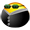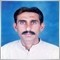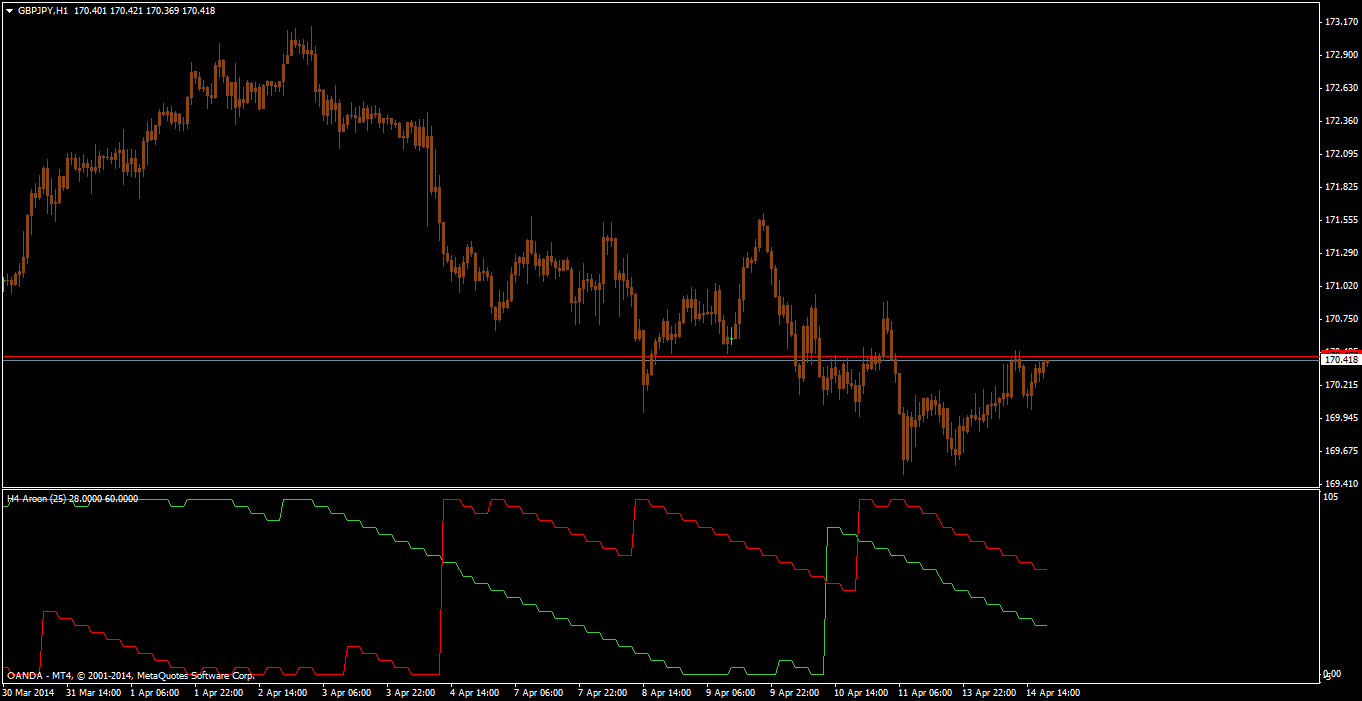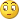# Indicators with alerts/signal - page 1343237

Hello , i'm looking to add alert to an indicator , help not forthcoming but still trying . I understand i have to find the SETINDEXBUFFER , in this case , the code of the indicator has 4 setindexbuffers , if i'm not wrong , now i am unable to find the buffer setting value in the indicator where i presumably have to add the following code ''Alert("Indicator set");}''to every buffer setting thereafter ...

Could anyone kind enough assist me in doing this ? I' seeing code for the first time in my life and it's hard to understand . Here is the code of the indicator

void init() {

IndicatorDigits(2);

SetIndexBuffer(0, g_ibuf_88);

SetIndexLabel(0, "LineaSqCD");

SetIndexStyle(0, DRAW_LINE, STYLE_SOLID, 3);

SetIndexBuffer(1, g_ibuf_92);

SetIndexLabel(1, "LineaSqCD");

SetIndexStyle(1, DRAW_LINE, STYLE_SOLID, 3);

SetIndexBuffer(2, g_ibuf_96);

SetIndexLabel(2, "LineaSqCD");

SetIndexStyle(2, DRAW_NONE);

SetIndexBuffer(3, g_ibuf_100);

SetIndexLabel(3, "LineaSqCD");

SetIndexStyle(3, DRAW_NONE);

Comment("");

}

int start() {

double ld_0;

double ld_8;

double ld_16;

double ld_24;

int li_44;

int li_48;

double l_ima_52;

int li_40 = IndicatorCounted();

if (li_40 >= 0) {

if (li_40 > 0) li_40--;

li_40 = Bars + 1 - li_40;

for (int li_36 = 0; li_36 < li_40; li_36++) {

g_ibuf_92[li_36] = -gi_84;

g_ibuf_88[li_36] = -gi_84;

l_ima_52 = iMA(NULL, 0, g_period_76, 0, MODE_EMA, PRICE_TYPICAL, li_36);

if (Close[li_36] > l_ima_52) g_ibuf_92[li_36] = EMPTY_VALUE;

if (Close[li_36] < l_ima_52) g_ibuf_88[li_36] = EMPTY_VALUE;

}

li_44 = Bars + 1 - gi_80 - 5;

li_48 = li_44 - gi_80 - 1;

for (li_36 = li_48; li_36 >= 0; li_36--) {

ld_0 = 0;

for (int li_32 = gi_80; li_32 >= 1; li_32--) {

ld_8 = gi_80 + 1;

ld_8 /= 3.0;

ld_16 = 0;

ld_16 = (li_32 - ld_8) * (Close[gi_80 - li_32 + li_36]);

ld_0 += ld_16;

}

ld_24 = 6.0 * ld_0 / (gi_80 * (gi_80 + 1));

g_ibuf_96[li_36] = gi_84;

g_ibuf_100[li_36] = gi_84;

if (ld_24 > Close[li_36]) g_ibuf_100[li_36] = EMPTY_VALUE;

if (ld_24 < Close[li_36]) g_ibuf_96[li_36] = EMPTY_VALUE;

}

}

return (0);

}2973

Stan007:
Hello , i'm looking to add alert to an indicator , help not forthcoming but still trying . I understand i have to find the SETINDEXBUFFER , in this case , the code of the indicator has 4 setindexbuffers , if i'm not wrong , now i am unable to find the buffer setting value in the indicator where i presumably have to add the following code ''Alert("Indicator set");}''to every buffer setting thereafter ...

Could anyone kind enough assist me in doing this ? I' seeing code for the first time in my life and it's hard to understand . Here is the code of the indicator

void init() {

IndicatorDigits(2);

SetIndexBuffer(0, g_ibuf_88);

SetIndexLabel(0, "LineaSqCD");

SetIndexStyle(0, DRAW_LINE, STYLE_SOLID, 3);

SetIndexBuffer(1, g_ibuf_92);

SetIndexLabel(1, "LineaSqCD");

SetIndexStyle(1, DRAW_LINE, STYLE_SOLID, 3);

SetIndexBuffer(2, g_ibuf_96);

SetIndexLabel(2, "LineaSqCD");

SetIndexStyle(2, DRAW_NONE);

SetIndexBuffer(3, g_ibuf_100);

SetIndexLabel(3, "LineaSqCD");

SetIndexStyle(3, DRAW_NONE);

Comment("");

}

int start() {

double ld_0;

double ld_8;

double ld_16;

double ld_24;

int li_44;

int li_48;

double l_ima_52;

int li_40 = IndicatorCounted();

if (li_40 >= 0) {

if (li_40 > 0) li_40--;

li_40 = Bars + 1 - li_40;

for (int li_36 = 0; li_36 < li_40; li_36++) {

g_ibuf_92[li_36] = -gi_84;

g_ibuf_88[li_36] = -gi_84;

l_ima_52 = iMA(NULL, 0, g_period_76, 0, MODE_EMA, PRICE_TYPICAL, li_36);

if (Close[li_36] > l_ima_52) g_ibuf_92[li_36] = EMPTY_VALUE;

if (Close[li_36] < l_ima_52) g_ibuf_88[li_36] = EMPTY_VALUE;

}

li_44 = Bars + 1 - gi_80 - 5;

li_48 = li_44 - gi_80 - 1;

for (li_36 = li_48; li_36 >= 0; li_36--) {

ld_0 = 0;

for (int li_32 = gi_80; li_32 >= 1; li_32--) {

ld_8 = gi_80 + 1;

ld_8 /= 3.0;

ld_16 = 0;

ld_16 = (li_32 - ld_8) * (Close[gi_80 - li_32 + li_36]);

ld_0 += ld_16;

}

ld_24 = 6.0 * ld_0 / (gi_80 * (gi_80 + 1));

g_ibuf_96[li_36] = gi_84;

g_ibuf_100[li_36] = gi_84;

if (ld_24 > Close[li_36]) g_ibuf_100[li_36] = EMPTY_VALUE;

if (ld_24 < Close[li_36]) g_ibuf_96[li_36] = EMPTY_VALUE;

}

}

return (0);

}

That is decompiled code237

Hi techmac , I actually didn't know it was decompiled , just found the thing on the internet . Being decompiled makes it impossible to code an alert into it ?154501

Stan007:
Hi techmac , I actually didn't know it was decompiled , just found the thing on the internet . Being decompiled makes it impossible to code an alert into it ?

Stan007

Being decompiled will make a lot of coders refuse to work on it (since decompiled code is a stolen code in 99.99% of cases)4412

Set the arrowsIdentifier parameter to unique value and set the arrow gap values to different values so that they are separated if they are displayed on the same bar

Thanks...the gap did it...1545014412

Then I guess well what happened to you(10 or more characters)19

When you mention those 10 or more characters are you refering to calling me DEGENERAT ?

That makes 9, you know!

You probably meant DEGENERATI, the Opus Dei sect's "Black Ops" M.I.B.4412

azorix:
When you mention those 10 or more characters are you refering to calling me DEGENERAT ?

That makes 9, you know!

You probably meant DEGENERATI, the Opus Dei sect's "Black Ops" M.I.B.I meant exactly what I wrote...тебя, чёрным-заражённым хуём, пидар.4549

mrtools:it seemed a correct version of aroon as you already informed only correct version of aroon reached at extreme values (-0,100) when others no.